# Octahedral group is isomorphic to S4

This article gives a proof/explanation of the equivalence of multiple definitions for the term symmetric group:S4
View a complete list of pages giving proofs of equivalence of definitions

## Statement

Consider the octahedral group, also known as a cube group. This is the group of all orientation-preserving self-isometries of$\R^3$ that send a particular regular octahedron to itself (different choices of such regular octahedra yield isomorphic groups). It can also be defined as the group of all orientation-preserving self-isometries of$\R^3$ that send a particular cube to itself.

This group is isomorphic to symmetric group:S4.

## Proof

### Why the octahedral group and the cube group are the same

The regular octahedron and the cube are dual Platonic solids. Explicitly, if we take the centers of the faces of a regular octahedron, we get a cube, and if we take the centers of the faces of a cube, we get a regular octahedron. Composing these processes (going from a regular octahedron to a cube and then again to a regular octahedron) gives a scaled version of the original. The process preserves isometry groups, i.e., the group for the original regular octahedron is the same as the group for the corresponding cube, and also the same as the group for the smaller regular octahedron.

### Construction of the homomorphism

The cube has eight vertices (these correspond to the eight faces of the regular octahedron). The vertices come in pairs of opposite vertices, and there are four such pairs. The midpoint of each such pair is the center of the cube.

Any element of the cube group must send a pair of opposite vertices to a pair of opposite vertices. Thus, we obtain a well-defined action of the cube group on the set of four pairs of opposite vertices. This group action defines a homomorphism from the cube group to symmetric group:S4. We will show that this homomorphism is an isomorphism.

### Injectivity

The kernel of the homomorphism comprises those orientation-preserving self-isometries of$\R^3$ that fix all four diagonals. We will show that this is trivial.

First, a note on the significance of "orientation-preserving": if we were allowed orientation-reversing transformations, then the map that sends each point to the point directly opposite it (with the center of the cube as their midpoint) would work as a nontrivial self-isometry that preserves all four diagonals.

With this idea in mind, we can work on a proof. The key insight behind the proof is that any self-isometry of$\R^3$ that fixes all four diagonals must either fix all vertices or flip all vertices (sending each to the one opposite it). This is easy to show: any vertex is adjacent (via an edge on the cube) to precisely one of the vertices for each of the other three diagonals, and is a face diagonal apart from the other vertex of those three diagonals. Therefore, if a particular vertex is fixed, all the three vertices adjacent to it must stay fixed in order to stay adjacent to it, and therefore, so must the vertices opposite them. Therefore, either all vertices are fixed, or all get flipped.

If all vertices get fixed, then, since the vertices affinely generate all of$\R^3$, the self-isometry must be the identity map. If all vertices get flipped, then the self-isometry matches, on all vertices, the self-isometry of$\R^3$ that sends each point to the opposite point with respect to the center of the cube. Since the vertices affinely generate$\R^3$, and the two self-isometries match on all points, they must be identical, so the self-isometry in question is the one that sends each point to the opposite point with respect to the center of the cube. This is an orientation-reversing isometry (its matrix is the negative identity matrix of order three, so its determinant is -1). Therefore, the only orientation-preserving self-isometry that fixes all four diagonals is the identity map, completing proof of injectivity.

### Surjectivity

There are two explicit styles of proof:

• For every permutation, describe how it arises from an orientation-preserving self-isometry.
• Show that all elements of a particular generating set of symmetric group:S4 arise from orientation-preserving self-isometries. For instance, we can use that transpositions generate the finitary symmetric group and then just show that all the transpositions arise from orientation-preserving self-isometries.

We will follow the former approach below, because it encompasses the latter and also provides more information about the group structure. Note that since we are limited to orientation-preserving self-isometries, these must all be rotations according to Euler's theorem; moreover, the angle of rotation should be$2\pi$ divided by the order of the element. Note that this is different from full tetrahedral group is isomorphic to S4 where only the even permutations are realized as rotations.

Partition Partition in grouped form Verbal description of cycle type Elements with the cycle type Size of conjugacy class Order of elements Even or odd? How it is obtained from an isometry Angle of rotation (should be$2\pi$ divided by the order) Numerical sanity check
1 + 1 + 1 + 1 1 (4 times) four cycles of size one each, i.e., four fixed points$()$ -- the identity element 1 1 even The identity map on$\R^3$.$2\pi$ or 0 -- depending on convention There's only 1 identity map so this checks out.
2 + 1 + 1 2 (1 time), 1 (2 times) one transposition (cycle of size two), two fixed points$(1,2)$,$(1,3)$,$(1,4)$,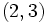$(2,3)$,$(2,4)$,$(3,4)$ 6 2 odd The half-turn (rotation by angle of$\pi$) about the line joining the midpoints of edges between pairs of vertices in the diagonals that we wish to interchange. The line joining the midpoints of the two edges is perpendicular to both of them.$\pi$ There are 12 edges, so 6 pairs of edges, so a total of 6 such rotations.
2 + 2 2 (2 times) double transposition: two cycles of size two$(1,2)(3,4)$,$(1,3)(2,4)$,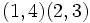$(1,4)(2,3)$ 3 2 even The half-turn (rotation by angle of$\pi$) about the line joining the midpoints of the pair of opposite faces such that on each face, the diagonally opposite vertices represent the two diagonals we want to interchange.$\pi$ There are 6 faces, so 3 pairs of opposite faces, matching the number of elements.
3 + 1 3 (1 time), 1 (1 time) one 3-cycle, one fixed point$(1,2,3)$,$(1,3,2)$,$(2,3,4)$,$(2,4,3)$,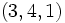$(3,4,1)$,$(3,1,4)$,$(4,1,2)$,$(4,2,1)$ 8 3 even Rotation by an angle$2\pi/3$ (clockwise or counter-clockwise, depending on the permutation we desire) about the axis of the diagonal that we wish to preserve.$2\pi/3$ There are 4 diagonals, and 2 rotations per diagonal, giving the total of 8.
4 4 (1 time) one 4-cycle, no fixed points$(1,2,3,4)$,$(1,2,4,3)$,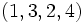$(1,3,2,4)$,$(1,3,4,2)$,$(1,4,2,3)$,$(1,4,3,2)$ 6 4 odd On the pair of opposite faces of the cube that have one vertex from each of the four diagonals in the proper cyclic order, a rotation by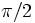$\pi/2$ in the proper direction (clockwise or counter-clockwise) that respects the cyclic order. The fixed axis of this rotation is the line joining the midpoints of the opposite faces.$\pi/2$ There are 6 faces, so 3 pairs of opposite faces, and for each face there are 2 rotations (clockwise and counter-clockwise) so there are 6 such rotations, matching the number of 4-cycles.